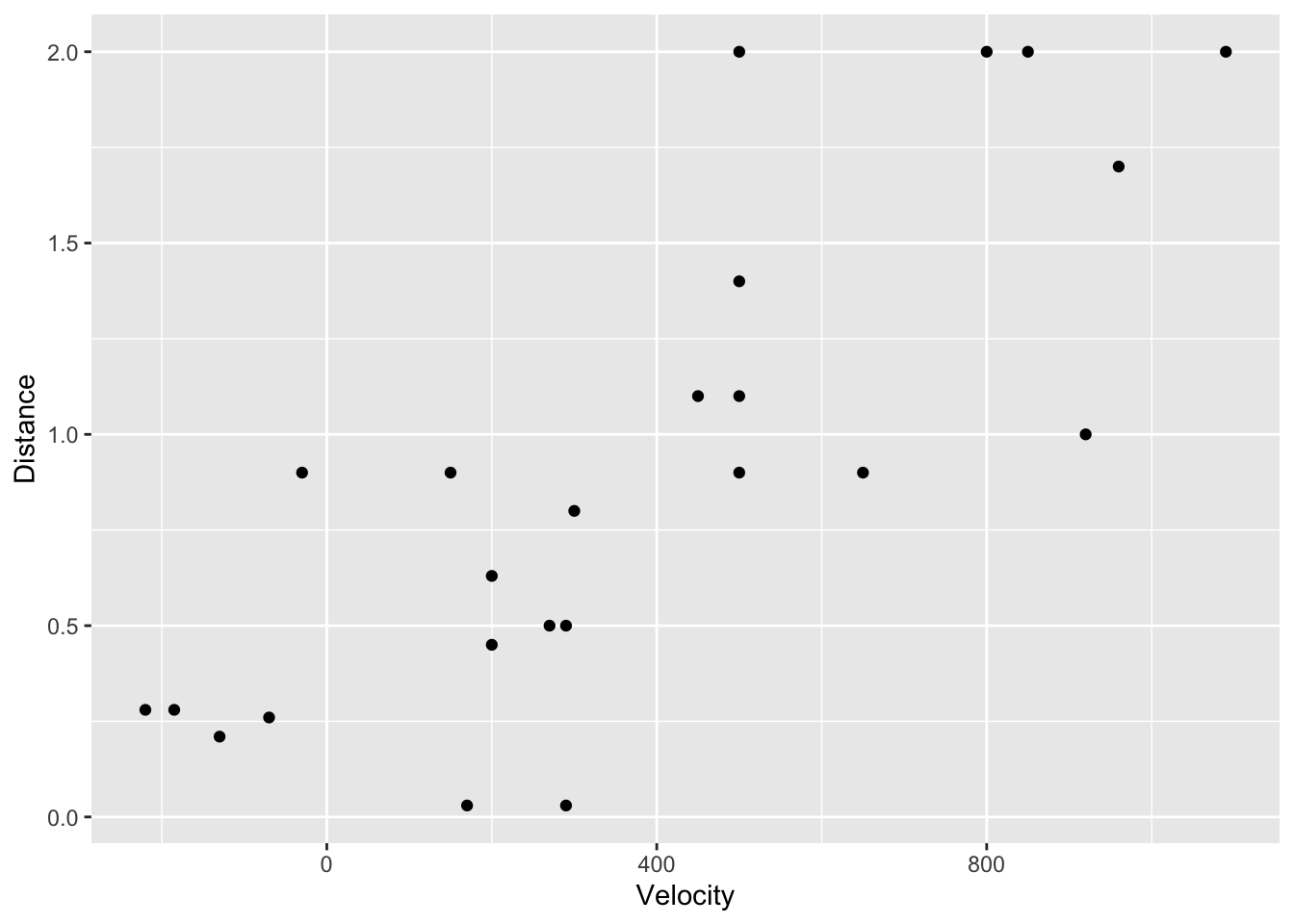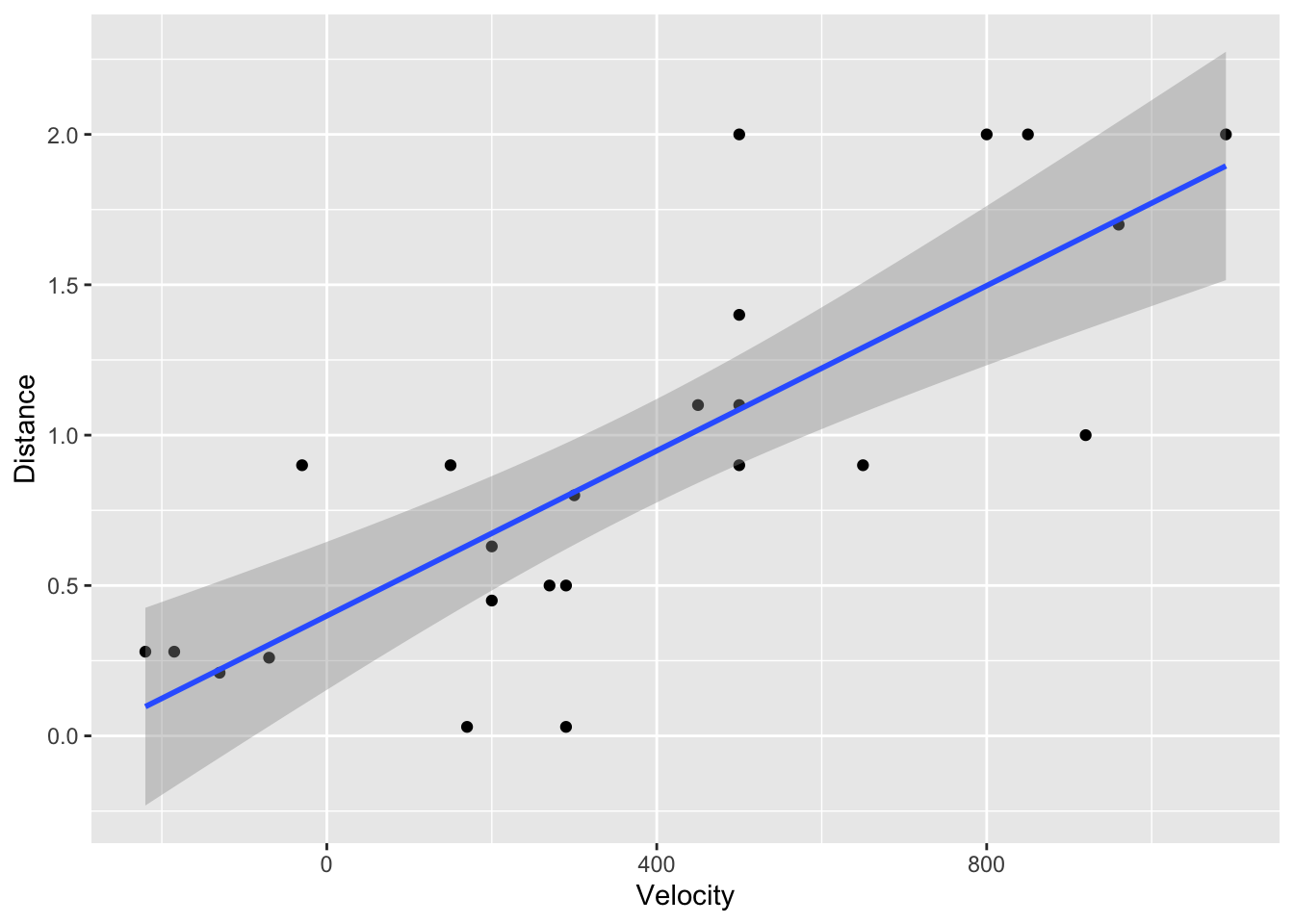In the code below, we will make use of the Sleuth3 package. To install the Sleuth3 package, use

install.packages("Sleuth3")

The installation step only needs to be done once, but then to use the package in an R session it needs to be loaded with the library() command as below.

library("tidyverse")
## ── Attaching packages ─────────────────────────────────────── tidyverse 1.3.0 ──
## ✓ ggplot2 3.3.2     ✓ purrr   0.3.4
## ✓ tibble  3.0.4     ✓ dplyr   1.0.2
## ✓ tidyr   1.1.2     ✓ stringr 1.4.0
## ✓ readr   1.4.0     ✓ forcats 0.5.0
## ── Conflicts ────────────────────────────────────────── tidyverse_conflicts() ──
## x dplyr::lag()    masks stats::lag()
library("Sleuth3")

options(scipen = 100) # eliminate scientific notation

## Simple linear regression

Simple linear regression involves two variables: independent variable and dependent variable. In this example, we will utilize data from the Hubble Telescope regarding the relationship between Velocity and Distance of 24 nebulae outside the Milky Way. Since the Distance depends on the recession Velocity, we will use Distance as the dependent variable and Velocity as the independent variable.

### Plots

The first step is to take a look at the data

head(case0701)
##   Velocity Distance
## 1      170     0.03
## 2      290     0.03
## 3     -130     0.21
## 4      -70     0.26
## 5     -185     0.28
## 6     -220     0.28
summary(case0701)
##     Velocity         Distance
##  Min.   :-220.0   Min.   :0.0300
##  1st Qu.: 165.0   1st Qu.:0.4075
##  Median : 295.0   Median :0.9000
##  Mean   : 373.1   Mean   :0.9113
##  3rd Qu.: 537.5   3rd Qu.:1.1750
##  Max.   :1090.0   Max.   :2.0000
ggplot(case0701, aes(x = Velocity, y = Distance)) +
geom_point()To add the regression line with uncertainty, we use

ggplot(case0701, aes(x = Velocity, y = Distance)) +
geom_point() +
stat_smooth(method = "lm", se = TRUE)
## geom_smooth() using formula 'y ~ x'From the help menu for these data, we find that the Velocity is measured in kilometers per second (km/s) and the Distance is measured in megaparsecs (Mpc).

### Run the regression

Recall that the simple linear regression model is $Y_i \stackrel{ind}{\sim} N(\beta_0+\beta_1X_i, \sigma^2).$ In this example, $$X_i$$ and $$Y_i$$ are the Velocity and Distance, respectively, for nebulae $$i$$.

To run the regression, we use

m <- lm(Distance ~ Velocity, data = case0701)

A summary of much of the regression analysis can be obtained using the summary() function.

summary(m)
##
## Call:
## lm(formula = Distance ~ Velocity, data = case0701)
##
## Residuals:
##      Min       1Q   Median       3Q      Max
## -0.76717 -0.23517 -0.01083  0.21081  0.91463
##
## Coefficients:
##              Estimate Std. Error t value   Pr(>|t|)
## (Intercept) 0.3991704  0.1186662   3.364     0.0028 **
## Velocity    0.0013724  0.0002278   6.024 0.00000461 ***
## ---
## Signif. codes:  0 '***' 0.001 '**' 0.01 '*' 0.05 '.' 0.1 ' ' 1
##
## Residual standard error: 0.4056 on 22 degrees of freedom
## Multiple R-squared:  0.6226, Adjusted R-squared:  0.6054
## F-statistic: 36.29 on 1 and 22 DF,  p-value: 0.000004608

#### Coefficients

To simply look at the estimated value of the coefficients.

coef(m)
## (Intercept)    Velocity
## 0.399170440 0.001372408

Here we have $$\hat\beta_0 = 0.4$$ and $$\hat\beta_1 = 0.0014$$. We can obtain 95% credible intervals for these two quantities using

confint(m)
##                    2.5 %     97.5 %
## (Intercept) 0.1530719058 0.64526897
## Velocity    0.0008999349 0.00184488

The interpretation for these parameters are - For a nebulae At a velocity of 0, the mean distance (Mpc) from Earth is 0.4 (0.15,0.65). - For each km/s increase in velocity, the mean distance (Mpc) from Earth increases by 0.0014 (0.0009,0.0018).

#### Estimate of the error standard deviation

The summary output also provides an estimate of the error standard deviation $$\sigma$$. In this exampe, it is estimated to be

summary(m)$sigma ##  0.4056302 #### Coefficient of determination The coefficient of determination, $$R^2$$, is interpreted as the proportion of variability in the dependent variable explained by this model. For these data, the coefficient of determination is summary(m)$r.squared
##  0.6225715

Often this value is multiplied by 100 to represent the results on a percentage basis.

Related to the coefficient of determination and regression is the correlation. The correlation is the square root of $$R^2$$ but you need to make sure the sign is consistent with the coefficient for the independent variable $$\beta_1$$. We can find the correlation directly using

cor(case0701$Velocity, case0701$Distance)
##  0.789032